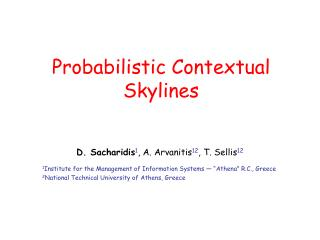Download PresentationProbabilistic Contextual Skylines

# Probabilistic Contextual Skylines - PowerPoint PPT PresentationDownload Presentation## Probabilistic Contextual Skylines

- - - - - - - - - - - - - - - - - - - - - - - - - - - E N D - - - - - - - - - - - - - - - - - - - - - - - - - - -
##### Presentation Transcript

1. Probabilistic Contextual Skylines D. Sacharidis1, A. Arvanitis12, T. Sellis12 1Institute for the Management of Information Systems — “Athena” R.C., Greece 2National Technical University of Athens, Greece

2. static vs. dynamic skyline • (another) hotels example • Price and Distance are Statically Preferred (SP) attributes with fixed preferences: lower is better • Ignore Amenity (assume all amenities are equally preferable) • h4 and h5 are in the static skyline • Amenity is a Relatively Preferred (RP) attribute, preferences are defined per query • h3, h4 andh5 are in the dynamic skyline

3. contextual skyline • just like dynamic skyline, but preferences are associated with some context • what if no preferences are specified for the current context? • two issues: • can we extract them from previous situations? • what does it mean to be in the skyline?

4. extract preferences • key idea is to combine preferences from similar contexts to the current • first assess the similarity between the current context Cq and all past contexts Cj : • contexts may have conflicting preferences, and we model uncertainty with probabilities • value u is better than v for the context Ci with some probability • probabilities can be extracted based on context similarities

5. probabilistic contextual skylines • dominance relationships are uncertain (assuming independence among attributes) • tuple t dominates t’ for the context Ci with probability the skyline probability of a tuple is defined as probabilistic contextual skyline query, p-CSQ, returns all tuples with

6. example (1) (2) (2) (3) skyline probability

7. non-indexed algorithms (1/2) • for RP attributes (unlike standard and tuple-probabilistic skylines) • nomonotonic visit order exists • transitivity is not preserved • we have to apply BNL-like methods (not SFS, BBS, etc.) • Basic Iterative Algorithm (BIA) • for each tuple scan the database and compute skyline probability (abort when below threshold)

8. non-indexed algorithms (2/2) • Candidate Selection Algorithm (CSA) • it identifies candidates • group tuples by their values on the RP attributes • tuples that are dominated in an RP-group have 0 probability • tuples that are in the skyline w.r.t. only the SP attributes have probability 1 CSA applies BIA only for the candidates (needs to check them against all tuples, though)

9. index-based algorithms (1/3) • the algorithms only consider the candidates • Basic Group Counting (BGC) • idea: tuples in an RP group that dominate a candidate t contribute the same probability • use COUNT aR-tree per RP group • but, don’t just issue a range query per tree… we don’t care about the exact count if tuple’s probability is below threshold • instead visit nodes from all trees in parallel and use a single priority queue • the node from the tree which has the highest expected probability of dominating tuple t has the largest priority

10. index-based algorithms (2/3) • Super Group Counting (SGC) • there can be a lot of RP groups with only a few tuples • to mitigate this, assign groups to super groups • use a GROUP-COUNT aR-tree per super-group • entry: < ei, MBBi, c[g1], c[g2], … > • where c[gj] is the number of tuples beneath node ei that belong to the j-th group • same algorithm as BGC… you only need to redefine the expected dominance probability to take into account multiple groups

11. index-based algorithms (3/3) • Batch Counting Algorithm (BCA) • all previous algorithms compute the skyline probability of one tuple at a time • BCA examines candidates in batches (as many as fit in memory) • extra bookkeeping with each heap entry to avoid double counting e1 is deheaped e1+ dominates t1 but not t2 entire e1 contributes to t1, but for t2 we need to expand e1 and enheap its children e2, e3 also remember e1+ with e2, e3

12. Experiments Non-indexed BIA, CSA Index-based SGC, BCA

13. Total Time vs. Dataset Cardinality Non-indexed BIA, CSA Index-based SGC, BCA

14. Total Time vs. RP domain size Non-indexed CSA Index-based SGC, BCA

15. Total Time vs. Dimensionality Non-indexed CSA Index-based SGC, BCA

16. thank you!